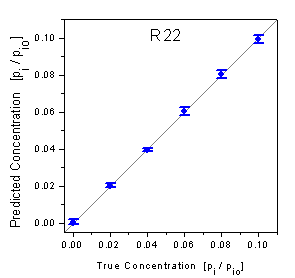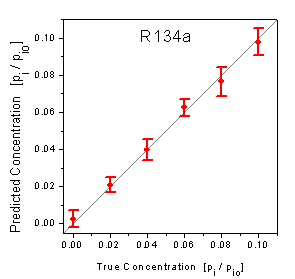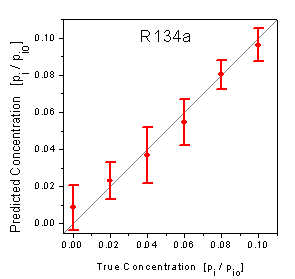Home News About Me Ph. D. Thesis Abstract Table of Contents 1. Introduction 2. Theory  Fundamentals of the Multivariate Data Analysis 3. Theory  Quantification of the Refrigerants R22 and R134a: Part I 3.1. Experimental 3.2. Single Analytes 3.3. Sensitivities 3.4. Calibrations of the Mixtures 3.5. Variable Selection by Brute Force 3.6. Conclusions 4. Experiments, Setups and Data Sets 5. Results  Kinetic Measurements 6. Results  Multivariate Calibrations 7. Results  Genetic Algorithm Framework 8. Results  Growing Neural Network Framework 9. Results  All Data Sets 10. Results  Various Aspects of the Frameworks and Measurements 11. Summary and Outlook 12. References 13. Acknowledgements Publications Research Tutorials Downloads and Links Contact Search Site Map Print this Page## 3.4.   Calibrations of the Mixtures

For the quantification of binary mixtures, neural networks were trained by the calibration data and subsequently predicted the independent test data. The root mean square errors (RMSE) of the test data and the crossvalidated calibration data measured with the 6-sensor array setup are listed in the first row of table 1. The RMSE of the prediction of the R134a concentrations is about three times higher than the RMSE of R22, whereby the relative RMSE of the test data are 3.5% for R22 and 13.0% for R134a. The RMSE of the test data of both analytes are only slightly higher than the RMSE of the crossvalidation of the calibration data indicating good calibration models . The predictions of the test data are graphically shown in the true-predicted plots in figure 9. Thereby the predicted concentrations of the test data are plotted versus the true known concentrations. Ideal predictions are consequently located on the diagonal. As the single predictions cannot be graphically resolved, the predictions of each concentration level are represented by the mean and the standard deviation. It is visible that the predictions of both analytes are not biased as the means of all concentration levels do not significantly deviate from the diagonal. The smaller standard deviations of the plot of R22 show that the predictions of the concentrations of R22 are more precise than the predictions of R134a. Both, the RMSE and the true predicted plots allow the conclusion that both, R22 and R134a, can be quantitatively determined in binary mixtures using the RIfS array setup. Especially the more important detection of the harmful R22 has proven to be very accurate over the whole concentration range.figure 9:    Predicted concentrations versus true concentrations of the test data measured by the array setup.figure 10:  Predicted concentrations versus true concentrations of the test data measured by the 4l setup.

The RMSE for the measurements performed by the 2-sensor 4l setup are listed in the second row of table 1, whereby the relative RMSE of the test data are 7.4% for R22 and 21.4% for R134a. The RMSE of the calibration data and the test data are also not significantly different indicating again the absence of typical problems of calibrations by neural networks like overtraining effects. Yet, the RMSE of both analytes are nearly twice as high as the RMSE of the measurements performed by the array setup with 6 sensors. The true-predicted plots in figure 10 correspond with this higher RMSE. The high standard deviations of the true-predicted plot of R134a show that the predictions are rather scattered. Additionally, the predictions of the low concentrations are a little biased with the absence of R134a being predicted as a relative saturation pressure of 0.0086 on average. On the other hand, the true-predicted plot of R22 shows that the predictions of R22 are not biased and more precise than the predictions of R134a demonstrating that a quantification of R22 in the presence of R134a is possible by using the low-cost 4l setup.

The deterioration of the quantification of both analytes using the 4l setup instead of the array setup raises the question, if this deterioration is caused by the reduction of the number of sensors or by the type of setup. Therefore, the data set recorded with the array setup was calibrated with neural networks again, but this time only the sensors PUT and UE2010 15% were used as input variables. For the comparison the UE 2010 15% layer is used instead of the 20% layer, as the UE 2010 layer used in the 4l setup is more similar to the UE 2010 15% layer in respect to the thickness of the layer. The RMSE of this experiment are listed in row 3 of table 1. The RMSE of R22 is nearly equal for the 4l setup and for the array setup with 2 sensors. Thus, the deterioration of the prediction of R22 can be primarily ascribed to the limitation of the number of sensors. On the other side, the RMSE of R134a of the 2-sensor array lies in between the 4l setup and the 6-sensor array. Hence, not only the limitation of the number of sensors, but also the type of setup plays a role for the quantification of R134a.

 Setup Crossv. R22 Crossv. R134a Test R22 Test R134a Test Mean Array: 6 Sensors 0.00162 0.00572 0.00183 0.00630 0.00406 4l: 2 Sensors 0.00325 0.01169 0.00317 0.01146 0.00732 Array: PUT + UE 2010 15% 0.00283 0.00750 0.00334 0.00891 0.00612 Array: PUT + UE 2010 20% 0.00182 0.00626 0.00203 0.00678 0.00440 Array: PDMS + UE 2010 20% 0.00184 0.00596 0.00206 0.00667 0.00436 Array: PDMS + M 2400 0.00238 0.00673 0.00268 0.00772 0.00520 Array: PDMS + UE 2010 15% 0.00276 0.00656 0.00329 0.00810 0.00569 Array: PUT + M 2400 0.00241 0.00776 0.00273 0.00881 0.00577 Array: PUT + PDMS 0.00611 0.01156 0.00685 0.01259 0.00972 Array: HBP + PDMS 0.0613 0.01122 0.00714 0.01257 0.00986 Array: HBP + UE 2010 20% 0.00177 0.01902 0.00199 0.02139 0.01169 Array: HBP + PUT 0.00657 0.01531 0.00773 0.01789 0.01281 Array: M 2400 + UE 2010 20% 0.00182 0.02371 0.00211 0.02371 0.01291 Array: HBP + UE 2010 15% 0.00316 0.02368 0.00384 0.02828 0.01606 Array: M 2400 + HBP 0.00320 0.02447 0.00393 0.02930 0.01661 Array: M 2400 + UE 2010 15% 0.00296 0.02774 0.00368 0.03436 0.01902 Array: UE 2010 20% +             UE 2010 15% 0.00187 0.02962 0.00210 0.03431 0.01821

table 1:      Root mean square errors for the calibration and prediction of the data measured by the array setup using 6 polymer sensors (1st row) and using all combinations for 2 sensors out of 6 sensors (3rd row to 17th row) . The root mean square errors for the miniaturized 4l setup, which uses 2 sensors, are listed in the 2nd row.

 Page 63 © Frank Dieterle, 03.03.2019